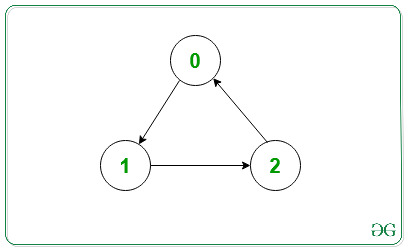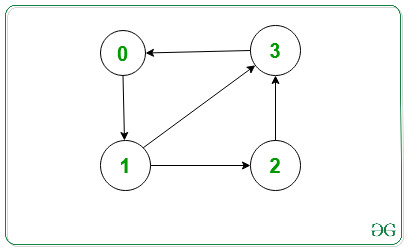Related Articles

# Print Adjacency List for a Directed Graph

• Last Updated : 09 Aug, 2021

An Adjacency List is used for representing graphs. Here, for every vertex in the graph, we have a list of all the other vertices which the particular vertex has an edge to.

Problem: Given the adjacency list and number of vertices and edges of a graph, the task is to represent the adjacency list for a directed graph.

Examples:

Input: V = 3, edges[][]= {{0, 1}, {1, 2} {2, 0}}Output:   0 -> 1
1 -> 2
2 -> 0
Explanation:
The output represents the adjacency list for the given graph.

Input: V = 4, edges[][] = {{0, 1}, {1, 2}, {1, 3}, {2, 3}, {3, 0}}Output:   0 -> 1
1 -> 2 3
2 -> 3
3 -> 0
Explanation:
The output represents the adjacency list for the given graph.

Approach (using STL): The main idea is to represent the graph as an array of vectors such that every vector represents the adjacency list of a single vertex. Using STL, the code becomes simpler and easier to understand.

Below is the implementation of the above approach:

## C++

 `// C++ program for the above approach` `#include ``using` `namespace` `std;` `// Function to add edges``void` `addEdge(vector<``int``> adj[], ``int` `u, ``int` `v)``{``    ``adj[u].push_back(v);``}` `// Function to print adjacency list``void` `adjacencylist(vector<``int``> adj[], ``int` `V)``{``    ``for` `(``int` `i = 0; i < V; i++) {``        ``cout << i << ``"->"``;``        ``for` `(``int``& x : adj[i]) {``            ``cout << x << ``" "``;``        ``}``        ``cout << endl;``    ``}``}` `// Function to initialize the adjacency list``// of the given graph``void` `initGraph(``int` `V, ``int` `edges, ``int` `noOfEdges)``{``    ``// To represent graph as adjacency list``    ``vector<``int``> adj[V];` `    ``// Traverse edges array and make edges``    ``for` `(``int` `i = 0; i < noOfEdges; i++) {` `        ``// Function call to make an edge``        ``addEdge(adj, edges[i], edges[i]);``    ``}` `    ``// Function Call to print adjacency list``    ``adjacencylist(adj, V);``}` `// Driver Code``int` `main()``{``    ``// Given vertices``    ``int` `V = 3;` `    ``// Given edges``    ``int` `edges = { { 0, 1 }, { 1, 2 }, { 2, 0 } };` `    ``int` `noOfEdges = 3;` `    ``// Function Call``    ``initGraph(V, edges, noOfEdges);` `    ``return` `0;``}`

## Java

 `// Java program for the above approach``import` `java.util.*;` `class` `GFG``{` `  ``// Function to add edges``  ``static` `void` `addEdge(Vector adj[], ``int` `u, ``int` `v)``  ``{``    ``adj[u].add(v);``  ``}` `  ``// Function to print adjacency list``  ``static` `void` `adjacencylist(Vector adj[], ``int` `V)``  ``{``    ``for` `(``int` `i = ``0``; i < V; i++) {``      ``System.out.print(i+ ``"->"``);``      ``for` `(``int` `x : adj[i]) {``        ``System.out.print(x+ ``" "``);``      ``}``      ``System.out.println();``    ``}``  ``}` `  ``// Function to initialize the adjacency list``  ``// of the given graph``  ``static` `void` `initGraph(``int` `V, ``int` `edges[][], ``int` `noOfEdges)``  ``{` `    ``// To represent graph as adjacency list``    ``@SuppressWarnings``(``"unchecked"``)``    ``Vector adj[] = ``new` `Vector[``3``];``    ``for``(``int` `i =``0``;i();``    ``}``    ``// Traverse edges array and make edges``    ``for` `(``int` `i = ``0``; i < noOfEdges; i++) {` `      ``// Function call to make an edge``      ``addEdge(adj, edges[i][``0``], edges[i][``1``]);``    ``}` `    ``// Function Call to print adjacency list``    ``adjacencylist(adj, V);``  ``}` `  ``// Driver Code``  ``public` `static` `void` `main(String[] args)``  ``{``    ``// Given vertices``    ``int` `V = ``3``;` `    ``// Given edges``    ``int` `edges[][] = { { ``0``, ``1` `}, { ``1``, ``2` `}, { ``2``, ``0` `} };` `    ``int` `noOfEdges = ``3``;` `    ``// Function Call``    ``initGraph(V, edges, noOfEdges);` `  ``}``}` `// This code is contributed by gauravrajput1`

## C#

 `// C# program for the above approach``using` `System;``using` `System.Collections.Generic;` `public` `class` `GFG``{` `  ``// Function to add edges``  ``static` `void` `addEdge(List<``int``> []adj, ``int` `u, ``int` `v)``  ``{``    ``adj[u].Add(v);``  ``}` `  ``// Function to print adjacency list``  ``static` `void` `adjacencylist(List<``int``> []adj, ``int` `V)``  ``{``    ``for` `(``int` `i = 0; i < V; i++) {``      ``Console.Write(i+ ``"->"``);``      ``foreach` `(``int` `x ``in` `adj[i]) {``        ``Console.Write(x+ ``" "``);``      ``}``      ``Console.WriteLine();``    ``}``  ``}` `  ``// Function to initialize the adjacency list``  ``// of the given graph``  ``static` `void` `initGraph(``int` `V, ``int` `[,]edges, ``int` `noOfEdges)``  ``{` `    ``// To represent graph as adjacency list  ``    ``List<``int``> []adj = ``new` `List<``int``>;``    ``for``(``int` `i = 0; i < adj.Length; i++) {``      ``adj[i] = ``new` `List<``int``>();``    ``}``    ` `    ``// Traverse edges array and make edges``    ``for` `(``int` `i = 0; i < noOfEdges; i++) {` `      ``// Function call to make an edge``      ``addEdge(adj, edges[i,0], edges[i,1]);``    ``}` `    ``// Function Call to print adjacency list``    ``adjacencylist(adj, V);``  ``}` `  ``// Driver Code``  ``public` `static` `void` `Main(String[] args)``  ``{``    ``// Given vertices``    ``int` `V = 3;` `    ``// Given edges``    ``int` `[,]edges = { { 0, 1 }, { 1, 2 }, { 2, 0 } };` `    ``int` `noOfEdges = 3;` `    ``// Function Call``    ``initGraph(V, edges, noOfEdges);` `  ``}``}` `// This code is contributed by Amit Katiyar`

Output:
```0->1
1->2
2->0```

Attention reader! Don’t stop learning now. Get hold of all the important DSA concepts with the DSA Self Paced Course at a student-friendly price and become industry ready.  To complete your preparation from learning a language to DS Algo and many more,  please refer Complete Interview Preparation Course.

In case you wish to attend live classes with experts, please refer DSA Live Classes for Working Professionals and Competitive Programming Live for Students.

My Personal Notes arrow_drop_up